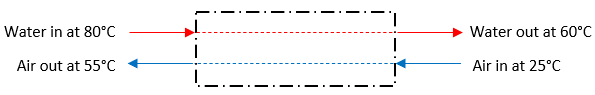## Counter-flow Heat Exchanger

In the below figure, some computer hardware components are water cooled via an insulated heat exchanger. If cold air is used to cool the hot water stream, what is the logarithmic-mean temperature difference for the heat exchanger? Assume the specific heats for both the water and air are constant. Note the specific heat (kJ/kg·K) for both water and air are 4.186 and 3.5 respectively.Hint
The Log Mean Temperature Difference (LMTD) for counter-flows in tubular heat exchangers:
$$\Delta T_{lm}=\frac{(T_{Ho}-T_{Ci})-(T_{Hi}-T_{Co})}{ln(\frac{T_{Ho}-T_{Ci}}{T_{Hi}-T_{Co}})}$$$where $$\Delta T_{lm}$$ is the log mean temperature difference, $$T_{Hi}$$ is the hot fluid’s inlet temperature, $$T_{Ho}$$ is the hot fluid’s outlet temperature, $$T_{Ci}$$ is the cold fluid’s inlet temperature, and $$T_{Co}$$ is the cold fluid’s outlet temperature. Hint 2 Specific heat is not needed to solve this problem. Because the problem statement has counter-flow streams, the Log Mean Temperature Difference (LMTD) in tubular heat exchangers is: $$\Delta T_{lm}=\frac{(T_{Ho}-T_{Ci})-(T_{Hi}-T_{Co})}{ln(\frac{T_{Ho}-T_{Ci}}{T_{Hi}-T_{Co}})}$$$
where $$\Delta T_{lm}$$ is the log mean temperature difference, $$T_{Hi}$$ is the hot fluid’s inlet temperature, $$T_{Ho}$$ is the hot fluid’s outlet temperature, $$T_{Ci}$$ is the cold fluid’s inlet temperature, and $$T_{Co}$$ is the cold fluid’s outlet temperature. Thus,
$$\Delta T_{lm}=\frac{(60^{\circ}C-25^{\circ}C)-(80^{\circ}C-55^{\circ}C)}{ln(\frac{60^{\circ}C-25^{\circ}C}{80^{\circ}C-55^{\circ}C})}$$$$$=\frac{35^{\circ}C-25^{\circ}C}{ln(\frac{35}{25})}=\frac{10^{\circ}C}{ln(1.4)}=\frac{10^{\circ}C}{0.336}=29.7^{\circ}C$$$
29.7°C